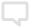隐私设置
3 TrembleiceTA的排名 101w+

### 算法导论学习记录 练习2.3-3 数学归纳法

1.解题思路1.根据题意，将2^ k代入n,使T(n) 变为 T(2^ k)，T(n+1)变为T(2^(k+1))2.代入解，若T(2^ (k+1))=2T((2^ (k+1))/2)+2^(k+1)成立，则可证明解成立。2.解题步骤设解成立，则有T(2k)=2^ klg(2^k)将2^ (k+1)代入n，则有T(2^ (k+1)) = 2T(2k) + 2^(k+1)依假设,T(2^ (k+1)) = 2^(k+1) * lg(2 ^ (k+1)).将T(2k)=2^ klg(2^k)代入T

2020-08-05 08:29:32

### 算法导论学习记录 2.1插入排序

1.插入排序有数组A[n] = {5,2,4,6,1,3}给出下标 j = 2 to n，表示正被插入到手中的’当前牌’。每次抽一次牌就进行一次由已排序数列的最大数向最小数的查找，查找过程用 i 作为当前被用于比较的牌的下标，对数列中的数依次查找。如果A[i] &gt; A[j]，则A[i+1] = A[i]，A[i] = A[j]，即将当前两个下标所指向的数交换位置，再将下标i向左退一位，实现数字在数组上的右移。直到循环结束，完成这一张牌的插入。已排序队列 A[1…j-1] 具有循环不变式的性

2020-07-31 02:48:12

•勤写标兵Lv1勤写标兵Lv1
授予每个自然周发布1篇到3篇原创IT博文的用户。本勋章将于次周周三上午根据用户上周的博文发布情况由系统自动颁发。